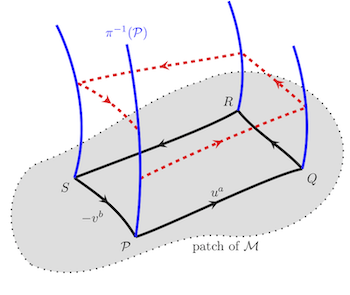# Research Theme: Gravitational-Wave Memory and Relativistic Angular Momentum

This post describes three papers related to defining a locally measured angular momentum, comparing this angular momentum measured by different observers, and understanding the relation of the observer dependence of these measurements to the gravitational-wave memory effect.

## Summary of the PapersBondi, Metzner, and Sachs (BMS) discovered that asymptotically flat spacetimes have an infinite-dimensional symmetry group, now called the BMS group, instead of the ten-dimensional symmetry group of flat spacetimes, the Poincare group. The additional symmetries not present in Minkowski spacetime are the so-called supertranslations, which are a type of angle-dependent translation around an isolated source. One important consequence is that in asymptotically flat spacetimes, there is not, in general, a preferred Poincare group with which one can define a special-relativistic angular momentum (except when the spacetime is stationary). Thus, observers who feign ignorance of the BMS group will notice that if they try to measure a type of special-relativistic angular momentum locally and compare their values with other observers, then their results will disagree. Thus, they would conclude that their notion of a special-relativistic angular momentum is observer dependent. But what would cause this observer dependence?

To investigate this question, we first defined a local procedure by which observers can measure special-relativistic angular momentum in stationary spacetimes. The algorithm uses just local measurements of the spacetime curvature and its gradients, and it gives the expected result in stationary spacetimes when measured by observers at large distances from the source. We then developed a method by which they can compare this angular momentum between different observers that takes into account the usual origin dependence of angular momentum in flat Minkowski space. The process of two observers comparing angular momentum at two different stationary points in a spacetime we could represent mathematically as a holonomy operation. By performing calculations involving a short-duration burst of gravitational waves passing by these observers, we determined that the changes in angular momentum of the spacetime would differ for the two observers. Moreover, we showed that in such a situation, the observer dependence is related to changes in the relative displacement of the observers that arise from the gravitational-wave memory effect.

Categories:

Updated: